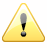## Flash与3D编程探秘（七）- 3D物体框架

#### 动画制作步骤

1. 首先在Flash IDE里绘制一个物体小P。

2. 开始设置还是和以前一样，原点，摄像机，焦距等等，另外不要忘记创建一个旋转角度object，存放物体在x，y和z轴的旋转角度变量。

// constants
var PI = 3.1415926535897932384626433832795;

// origin is the center of the view point in 3d space
// everything scale around this point
// these lines of code will shift 3d space origin to the center
var origin = new Object();
origin.x
= stage.stageWidth/2;
origin.y
= stage.stageHeight/2;
origin.z
= 0;

// focal length of viewer's camera
var focal_length = 300;

// now create a scene object to hold the spinning box
var scene = new Sprite();
scene.x
= origin.x;
scene.y
= origin.y
this.addChild(scene);

var axis_rotation
= new Object();
axis_rotation.x
= 0;
axis_rotation.y
= 0;
axis_rotation.z
= 0;

var camera
= new Object();
camera.x
= 0;
camera.y
= 0;
camera.z
= 0;

3. 写一个函数，用它来创建空间中的一个点，scale_point代表这个点在投射到2D平面上后位置缩放的比率。

// this function construct a 3d vertex
function vertex3d(x, y, z, scale = 1):Object
{
var point3d
= new Object();
point3d.x
= x;
point3d.y
= y;
point3d.z
= z;
point3d.scale_point
= scale;

return point3d;
}

4. 下面发挥一下你的空间想象力，使用第3步的函数创建正方体的8个顶点，并且把它们添加到一个数组里。

// we calculate all the vertex
var len = 50;                    // half of the cube width
// now create the vertexes for the cube
var points = [

//        x        y        z
vertex3d(-len,    -len,     -len),            // rear upper left
vertex3d(len,    -len,     -len),            // rear upper right
vertex3d(len,    -len,     len),            // front upper right
vertex3d(-len,    -len,     len),            // front upper left

vertex3d(
-len,    len,     -len),            // rear lower left
vertex3d(len,    len,     -len),            // rear lower right
vertex3d(len,    len,     len),            // front lower right
vertex3d(-len,    len,     len),            // front lower left
];

5. 初始化8个小P，并且把它们放在8个顶点（映射到xy轴上的点）所在的x和y位置。

// init balls and put them on the screen
for (var i = 0; i < points.length; i++)
{
var ball
= new Sphere();
ball.x
= points[i].x;
ball.y
= points[i].y;
ball.z
= 0;
scene.addChild(ball);
}

6. 这个函数你在摄像机空间旋转一篇文章中应该见过，函数的功能是把3D空间的点，映射到2D平面xy上。函数执行的步骤是这样的：

a) 提前计算出x，y和z旋转角度的正余弦值。
b) 使用for loop遍历物体所有的顶点。
c) 使用计算出的正余弦和三角函数对三个轴的旋转分别进行计算，得出旋转后顶点的x，y和z。
d) 然后计算出物体在2D平面上映射后的x和y值。
e) 并且把这些2D点添加到一个新的数组里。

f) 最后返回这个数组。

function project_pts(points)
{
var projected
= [];

// declare some variable for saving function call
var sin_x = Math.sin(axis_rotation.x);
var cos_x
= Math.cos(axis_rotation.x);
var sin_y
= Math.sin(axis_rotation.y);
var cos_y
= Math.cos(axis_rotation.y);
var sin_z
= Math.sin(axis_rotation.z);
var cos_z
= Math.cos(axis_rotation.z);

var x, y, z,
// 3d x, y, z
xy, xz,            // rotate about x axis
yx, yz,            // rotate about y axis
zx, zy,            // rotate about z axis
scale;            // 2d scale transform

for (var i = 0; i < points.length; i++)
{
x
= points[i].x;
y
= points[i].y;
z
= points[i].z;

// here is the theroy:

// suppose a is the current angle, based on given current_x, current_y on a plane

// (can be x, y plane, or y, z plane or z, x plane), rotate angle b

// then the new x would be radius*cos(a+b) and y would be  radius*sin(a+b)

// radius*cos(a+b) = radius*cos(a)*cos(b) - radius*sin(a)*sin(b)

// radius*sin(a+b) = radius*sin(a)*cos(b) + radius*cos(a)*sin(b)

// rotate about x axis
xy = cos_x*- sin_x*z;
xz
= sin_x*+ cos_x*z;

// rotate about y axis
yz = cos_y*xz - sin_y*x;
yx
= sin_y*xz + cos_y*x;

// rotate about z axis
zx = cos_z*yx - sin_z*xy;
zy
= sin_z*yx + cos_z*xy;

// scale it
scale = focal_length/(focal_length+yz-camera.z);
x
= zx*scale - camera.x;                // get x position in the view of camera
y = zy*scale - camera.y;                // get x position in the view of camera

projected[i]
= vertex3d(x, y, yz, scale);
}

return projected;
}

7. 下面是动画执行的循环函数。需要注意的一点，在以后的文章中我都将使用基于时间的运动。在这里你只要知道下面的公式就可以了：旋转角度=角速度X时间。使用上面的公式，递增物体围绕y轴和x轴的旋转角度。然后使用第6步的函数计算所有的3D顶点旋转后的位置并且得到映射后的2D点。剩下你应该能想到，就是把相应的小P定位到这些定点上，并且对小球进行缩放比率scale_point，最后不要忘记对小P进行z排序。

function move(e:Event):void
{

// well we use time based movement in this tutorial
var current_time = new Date().getTime();                // sampe the current time

// increment the rotation around y axis
axis_rotation.y += 0.0008*(current_time-start_time);

// increment the rotation around x axis
axis_rotation.x += 0.0006*(current_time-start_time);
start_time
= current_time;                                // reset the start time

var projected
= project_pts(points);        // 3d ponts to 2d transformation

// now we have all the data we need to position the balls
for (var i = 0; i < scene.numChildren; i++)                // loop throught the scene
{

// positioning the ball
scene.getChildAt(i).x = projected[i].x;
scene.getChildAt(i).y
= projected[i].y;
scene.getChildAt(i).z
= projected[i].z;
scene.getChildAt(i).scaleX
= scene.getChildAt(i).scaleY = projected[i].scale_point;
}

swap_depth(scene);
// sort out the depth
}

// bubble sort algo
function swap_depth(container:Sprite)
{

for (var i = 0; i < container.numChildren - 1; i++)
{

for (var j = container.numChildren - 1; j > 0; j--)
{

if (Object(container.getChildAt(j-1)).z < Object(container.getChildAt(j)).z)
{
container.swapChildren(container.getChildAt(j
-1), container.getChildAt(j));
}
}
}
}

// now add the event listener and spin the box
this.addEventListener(Event.ENTER_FRAME, move);

#### 制作步骤

1. 基本上的代码和前面是一样的，同样需要设置场景，创建正方体的顶点，注意不要再在舞台上添加小P。

2. 当把3D点映射到2D平面上后，使用黑线把正方体相邻的两个点连接起来。非常容易理解，不过注意，有很多种办法连接这些点，你可以先连接正方体顶面的点，然后底面的点，最后连接顶面和底面，不要局限于这几种，试着去发现新的方法，找到合适你思维方式的一种。

function move(e:Event):void
{

// well we use time based movement in this tutorial
var current_time = new Date().getTime();                // sampe the current time

// increment the rotation around y axis
axis_rotation.y += 0.0008*(current_time-start_time);

// increment the rotation around x axis
axis_rotation.x += 0.0006*(current_time-start_time);
start_time
= current_time;                            // reset the start time

var projected
= project_pts(points);        // 3d ponts to 2d transformation

// now we start drawing the cube
with (scene.graphics)
{
clear();
lineStyle(
0.50x0F6F9F1);

// top face
moveTo(projected.x, projected.y);
lineTo(projected[
1].x, projected.y);
lineTo(projected[
2].x, projected.y);
lineTo(projected[
3].x, projected.y);
lineTo(projected[
0].x, projected.y);

// bottom face
moveTo(projected.x, projected.y);
lineTo(projected[
5].x, projected.y);
lineTo(projected[
6].x, projected.y);
lineTo(projected[
7].x, projected.y);
lineTo(projected[
4].x, projected.y);

// vertical lines
moveTo(projected.x, projected.y);
lineTo(projected[
4].x, projected.y);
moveTo(projected[
1].x, projected.y);
lineTo(projected[
5].x, projected.y);
moveTo(projected[
2].x, projected.y);
lineTo(projected[
6].x, projected.y);
moveTo(projected[
3].x, projected.y);
lineTo(projected[
7].x, projected.y);
}
}

3. 还有一个地方需要改动，因为不再对顶点的物体进行缩放，所以就必须要传递scale_point这个属性。

// this function construct a 3d vertex
function vertex3d(x, y, z):Object
{
var point3d
= new Object();
point3d.x
= x;
point3d.y
= y;
point3d.z
= z;

return point3d;
}

DNA链

#### 关于Time Based和Frame Based运动作者：Yang Zhou 出处：http://yangzhou1030.cnblogs.com 本文版权归作者和博客园共有，转载未经作者同意必须保留此段声明。请在文章页面明显位置给出原文连接，作者保留追究法律责任的权利。

posted on 2008-11-09 13:08 yangzhou1030 阅读(...) 评论(...) 编辑 收藏